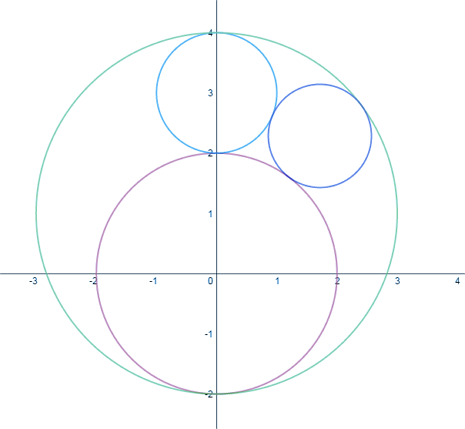# Shocked Face

Geometry Level 5Let $R$ be the region in the first quadrant outside of the circles $x^{2} + y^{2} = 4$ and $x^{2} + (y - 3)^{2} = 1$ but inside the circle $x^{2} + (y - 1)^{2} = 9.$

The largest circle that can be inscribed in $R$ has radius $\dfrac{a}{b}$, where $a$ and $b$ are positive coprime integers. Find $a + b$.

×

Problem Loading...

Note Loading...

Set Loading...